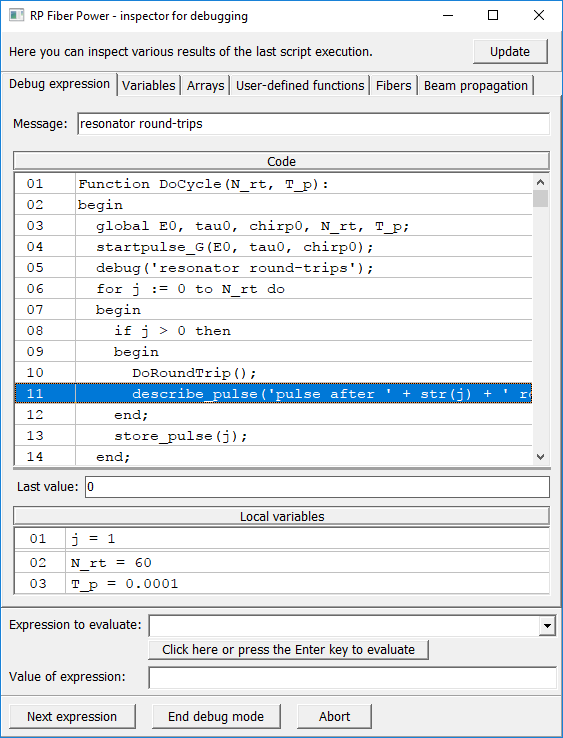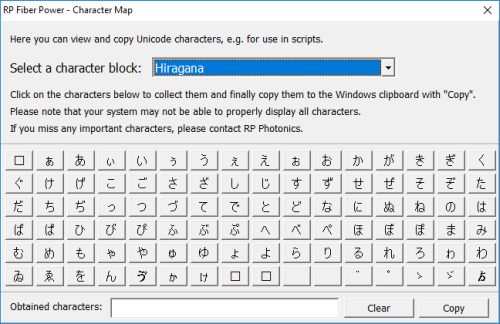## RP系列 | 强大的调试器和软件新功能

2021-05-26 16:01发布`if alpha > 0 thenbegin  sum := 0  for j := 1 to N do    sum := sum + f(j, alpha)  show "Result: ", sum:d3end`

`for j:=1 to Ndo sum:=sum+f(j,alpha)`

I would now write:

`for j := 1 to N do  sum := sum + f(j, alpha)`